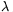Mathematical and Physical Journal
for High Schools
Issued by the MATFUND Foundation
 Already signed up? New to KöMaL?

#Problem A. 379. (September 2005)

A. 379. Find all real numbersfor which there exists a non-zero polynomial P, such thatfor all n. List all such polynomials for=2.

(5 pont)

Deadline expired on October 17, 2005.

Sketch of solution. Let P(x)=a0+a1x+...+akxk. Both sides of the equation contains a polynomial of n, degree is k. The coefficients must pairwise match.

Sincethe coefficient of nk ison the left-hand side, and it isak on the right-hand side. Therefore,.

Ifthen we have a homogenous system of linear equations for the coefficients and one of these equations vanishes. So the system has infinitely many solutions.

For=2 we obtain k=3 and P(x)=c(x3-x).

### Statistics:

 15 students sent a solution. 5 points: Erdélyi Márton, Estélyi István, Gyenizse Gergő, Kónya 495 Gábor, Nagy 224 Csaba, Paulin Roland, Tomon István. 4 points: Jankó Zsuzsanna, Molnár 999 András. 3 points: 2 students. 1 point: 1 student. 0 point: 3 students.

Problems in Mathematics of KöMaL, September 2005